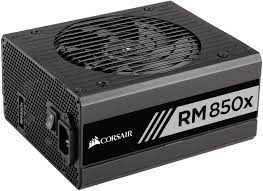## Power Units

What are the base SI units for power?Hint
Power is the time rate of doing work. The unit for power is a watt (W).
Power is the time rate of doing work. The unit for power is a watt (W), which is one joule per second.
$$1\:W=1\:\frac{J}{s}$$$Joule (J) is a unit of work, which is the product of a unit force (one newton) acting through a unit distance (one meter). $$1\:J=1\:N\cdot m$$$
A newton is a unit of force, which is described in the following SI base units:
$$\frac{m\cdot kg}{s^{2}}$$$Combining all units together: $$W=\frac{J}{s}=\frac{N\cdot m}{s}=\frac{m^2\cdot kg}{s^{3}}$$$
(A familiar unit for power in English units is horsepower (hp), where $$1\:hp=550\:\frac{ft\cdot lbf}{s}$$ )
$$\frac{m^2\cdot kg}{s^{3}}$$\$